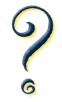Definition of

# ProblemA question that needs a solution.

In mathematics some problems use words:

"John was traveling at 20 km per hour for half an hour. How far did he travel?"

And some use equations:

"Solve x+5=22"